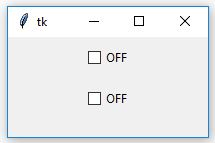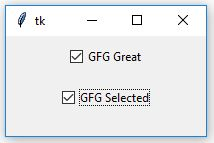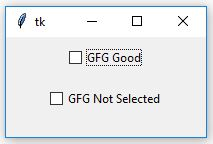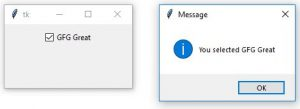# Python | How to dynamically change text of Checkbutton

Tkinter is a GUI (Graphical User interface) module which is used to create various types of applications. It comes along with the Python and consists of various types of widgets which can be used to make GUI more attractive and user-friendly. Checkbutton is one of the widgets which is used to select multiple options.

Checkbutton can be created as follows:

`chkbtn = ttk.Checkbutton(parent, value = options, ...)`

Code #1:

 `# This will import tkinter and ttk ` `from` `tkinter ``import` `*` `from` `tkinter ``import` `ttk ` ` `  `root ``=` `Tk() ` ` `  `# This will set the geometry to 200x100 ` `root.geometry(``'200x100'``) ` ` `  `text1 ``=` `StringVar() ` `text2 ``=` `StringVar() ` ` `  `# These text are used to set initial ` `# values of Checkbutton to off ` `text1.``set``(``'OFF'``) ` `text2.``set``(``'OFF'``) ` ` `  `chkbtn1 ``=` `ttk.Checkbutton(root, textvariable ``=` `text1, variable ``=` `text1, ` `                          ``offvalue ``=` `'GFG Not Selected'``, ` `                          ``onvalue ``=` `'GFG Selected'``) ` ` `  `chkbtn1.pack(side ``=` `TOP, pady ``=` `10``) ` `chkbtn2 ``=` `ttk.Checkbutton(root, textvariable ``=` `text2, variable ``=` `text2, ` `                          ``offvalue ``=` `'GFG Average'``, ` `                          ``onvalue ``=` `'GFG Good'``) ` `chkbtn2.pack(side ``=` `TOP, pady ``=` `10``) ` ` `  `root.mainloop() `

Output #1: When you run application you see the initial states of Checkbutton as shown in output.Output #2: As soon as you select the Checkbutton you’ll see that text has been changed as in output.Output #3: When you deselect the Checkbutton you’ll again observe following changes.Code #2: Commands can be integrate with the Checkbutton which can be execute when checkbutton is selected or deselected depending upon conditions.

 `# Importing tkinter, ttk and ` `# _show method to display ` `# pop-up message window ` `from` `tkinter ``import` `*` `from` `tkinter ``import` `ttk ` `from` `tkinter.messagebox ``import` `_show ` ` `  `root ``=` `Tk() ` `root.geometry(``'200x100'``) ` ` `  `text1 ``=` `StringVar() ` `text1.``set``(``'OFF'``) ` ` `  `# This function is used to display ` `# the pop-up message ` `def` `show(event): ` `    ``string ``=` `event.get() ` `    ``_show(``'Message'``, ``'You selected '` `+` `string) ` ` `  `chkbtn1 ``=` `ttk.Checkbutton(root, textvariable ``=` `text1, variable ``=` `text1, ` `                          ``offvalue ``=` `'GFG Good'``, ` `                          ``onvalue ``=` `'GFG Great'``, ` `                          ``command ``=` `lambda` `: show(text1)) ` `chkbtn1.pack(side ``=` `TOP, pady ``=` `10``) ` ` `  `root.mainloop() `

Output:Note: In above code offvalue and onvalue are used to set the values of Checkbutton of non-selected state and selected state respectively.

Whether you're preparing for your first job interview or aiming to upskill in this ever-evolving tech landscape, GeeksforGeeks Courses are your key to success. We provide top-quality content at affordable prices, all geared towards accelerating your growth in a time-bound manner. Join the millions we've already empowered, and we're here to do the same for you. Don't miss out - check it out now!

Previous
Next